## Suppose a tank contains 400 gallons of salt water. If pure water flows into the tank at the rate of 7 gallons per minute and the mixture flo

Question

Suppose a tank contains 400 gallons of salt water. If pure water flows into the tank at the rate of 7 gallons per minute and the mixture flows out at the rate of 3 gallons per minute, how many pounds of salt will remain in the tank after 16 minutes if 28 pounds of salt are in the mixture initially? (Give your answer correct to at least three decimal places.)

in progress 0
2 months 2021-07-23T12:15:38+00:00 1 Answers 0 views 0

Step-by-step explanation:

This is a differential equation problem most easily solved with an exponential decay equation of the form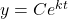. We know that the initial amount of salt in the tank is 28 pounds, so

C = 28. Now we just need to find k.

The concentration of salt changes as the pure water flows in and the salt water flows out. So the change in concentration, where y is the concentration of salt in the tank, is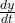. Thus, the change in the concentration of salt is found in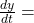inflow of salt – outflow of salt

Pure water, what is flowing into the tank, has no salt in it at all; and since we don’t know how much salt is leaving (our unknown, basically), the outflow at 3 gal/min is 3 times the amount of salt leaving out of the 400 gallons of salt water at time t: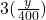Therefore,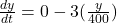or just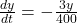and in terms of time,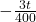Thus, our equation is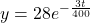and filling in 16 for the number of minutes in t:

y = 24.834 pounds of salt